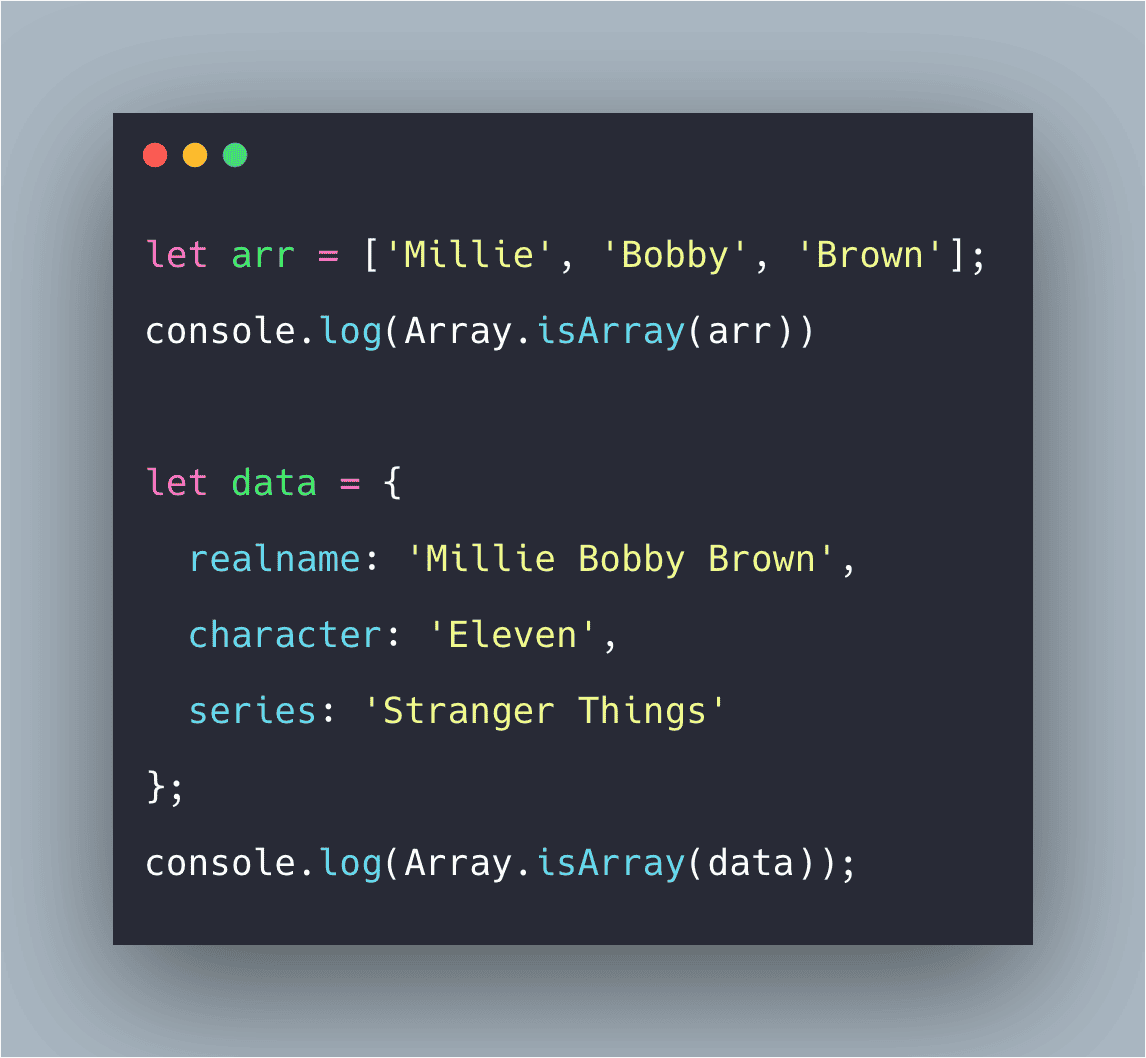# Javascript Array isArray Tutorial With Example | Array.isArray() FunctionThe Array.isArray() method determines whether the passed value is the Array. If the value is the Array, then true is returned; otherwise, false is return.

Javascript array isArray() is an inbuilt function that determines whether the passed value is an array or not. If the value is an array, then true is returned; otherwise, false is return. When checking for the Array instance, the isArray() *function is preferred over the *instanceof because it works through iframes.

So, if you want to check if the input value is an  array or not then we can use the Javascript isArray() function.

There are some use cases like let say, we are fetching the json data from the server and then we convert it into an  object and then if we wanted to make sure that the data object contains the array values or not then we can use the isArray() function.

We can check the data type of any value of Javascript using  typeof operator.

## Javascript Array isArray Tutorial With Example | Array.isArray() Function

The Array.isArray() method determines whether the passed value is the Array. If the value is the Array, then true is returned; otherwise, false is return.

## Javascript Array lastIndexOf Example | JS Array lastIndexOf()

Javascript Array lastIndexOf Tutorial | JS Array lastIndexOf() Example. A lastIndexOf() method searches an array for a specified item and returns its index.

## Javascript Array lastIndexOf Example | JS Array lastIndexOf()

Javascript Array lastIndexOf Tutorial | JS Array lastIndexOf() Example. A lastIndexOf() method searches an array for a specified item and returns its index.

## Javascript Array copyWithin Example | JS Array copyWithin

Javascript Array copyWithin() is an inbuilt method that copies part of an array to the same array and returns it, without modifying its size i.e, copies array element of an array within the same array.

## Javascript Array copyWithin Example | JS Array copyWithin

Javascript Array copyWithin() is an inbuilt method that copies part of an array to the same array and returns it, without modifying its size i.e, copies array element of an array within the same array.